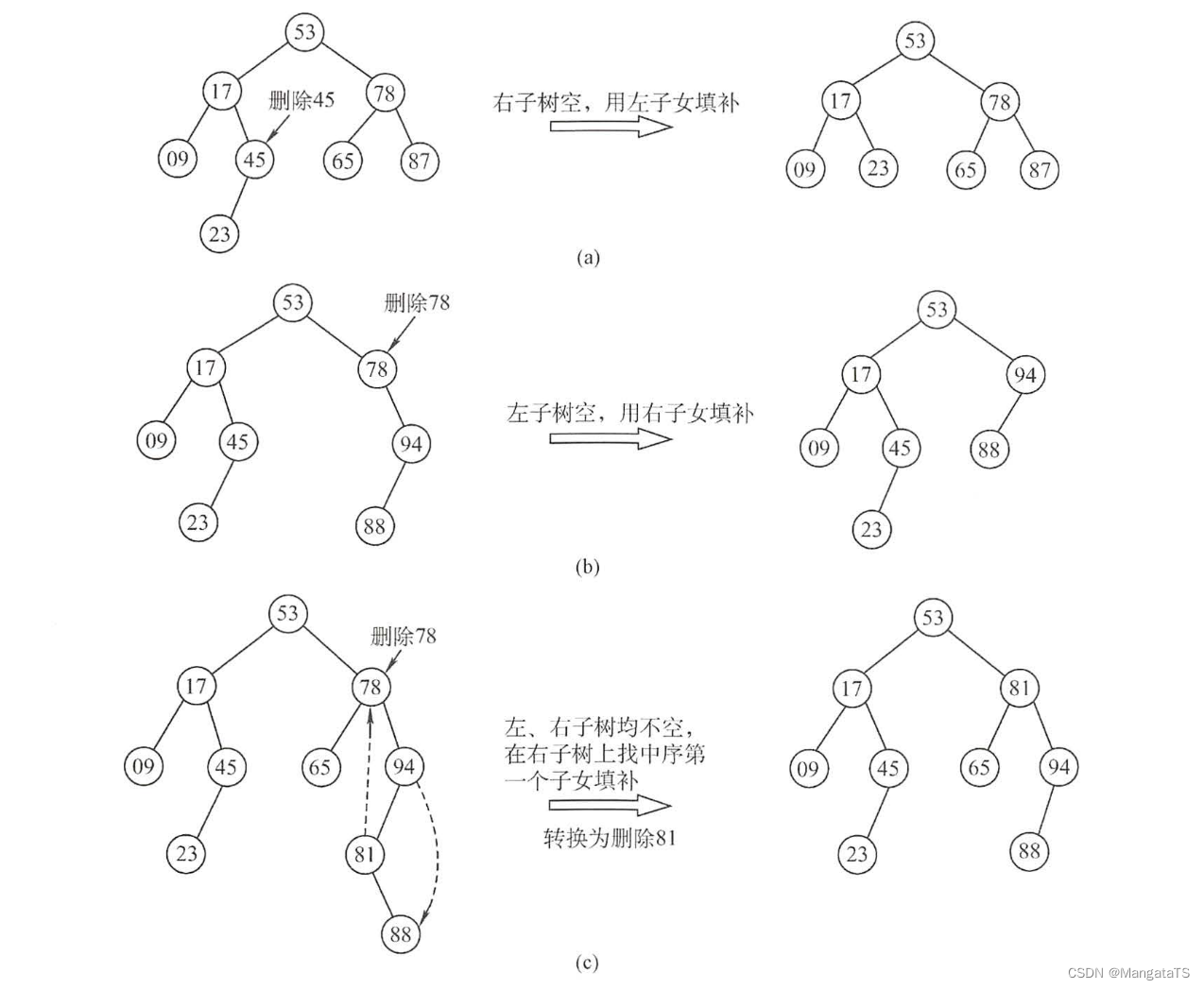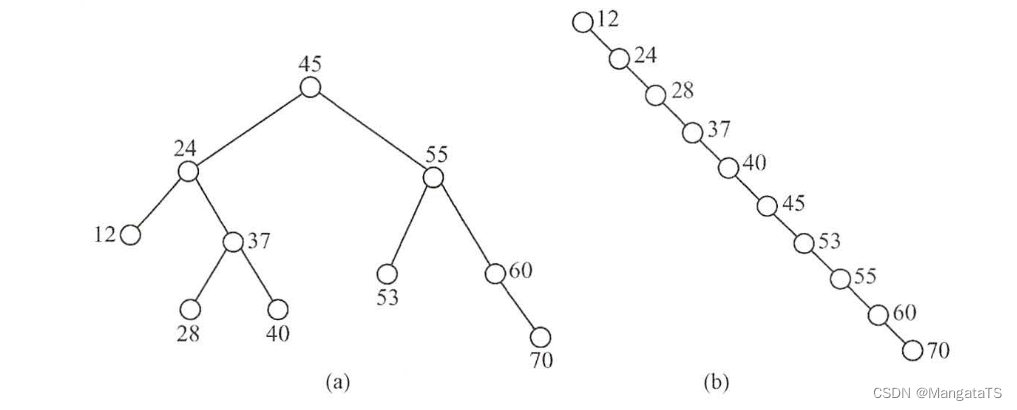# 二叉排序树¶

## 一、定义¶

• 若左子树非空，则左子树上所有结点的值均小于根结点的值。
• 若右子树非空， 则右子树上所有结点的值均大于根结点的值
• 左、右子树也分别是一棵二叉排序树。

typedef struct Node{
ElemType data;
struct Node *lchild, *rchild;
}Node;


• 其二（用于竞赛代码）
struct Node{
int val,ls,rs,cnt;//分别表示的是结点的值、左儿子、右儿子、结点出现的次数
}tree;


## 二、 查找操作¶

• case1

Node *BST_Search(Node *root,ElemType key) {
while(root != NULL && root->data != key) {
if(root->data < key) root = root->rchild;
else root = root->lchild;
}
return root;
}


• case2

int find(int x,int v)//x是当前查询位置的下标，v是查询的值
{
while(x != 0 && tree[x].val != v) {
if(tree[x].val < v) x = tree[x].ls;//往左走
else x = tree[x].rs;//往右走
}
if(tree[x].cnt == 0) x = -1;
return x;
}


## 三、插入操作¶

• case1

Node * Create_Node(ElemType key) {
Node *p = (Node)malloc(sizeof(Node));
p->data = key;
p->lchild = p->rchild = NULL;
}

int BST_insert(Node *root,ElemType key) {
if(!root) {//如果是根节点元素为空的话
root = Create_Node(key);
return 1;
}
if(root->data == key)
return 0;//已经存在，插入失败
else if(root->data < key) {//插入到右子树
if(root->rchild == NULL) {
Node *p = Create_Node(key);
root->rchild = p;
return 1;//成功插入
} else {
return BST_insert(root->rchild,key);
}
}
else {//插入到左子树
if(root->lchild == NULL) {
Node *p = Create_Node(key);
root->lchild = p;
return 1;//成功插入
} else {
return BST_insert(root->lchild,key);
}
}
}


• case2

void add(int x,int v)//x是当前查询位置的下标，v是插入的值
{
if(tree[x].val==v){
//如果恰好有重复的数，就把cnt++，退出即可，因为我们要满足第四条性质
tree[x].cnt++;
return ;
}
if(tree[x].val>v){//如果v<tree[x].val，说明v实在x的左子树里
if(tree[x].ls!=0)
else{//如果不是，v就是x的左子树的权值
cont++;//cont是目前BST一共有几个节点
tree[cont].val=v;
tree[x].ls=cont;
}
}
else{//右子树同理
if(tree[x].rs!=0)
else{
cont++;
tree[cont].val=v;
tree[x].rs=cont;
}
}
}


## 四、构造操作¶

• case1
Node *Create_BST(Node *root,ElemType vec[],int n) {
root = NULL;
for(int i = 0;i < n; ++i) {
BST_insert(root,vec[i]);
}
return root;
}

• case2
int Create_BST(int root,vector<int> vec,int n) {
root = 1;
for(int i = 0;i < n; ++i) {
}
return root;
}


## 五、删除操作¶

• ①若被删除结点 $$z$$ 是叶结点，则直接删除，不会破坏二叉排序树的性质
• ②若结点 $$z$$ 只有一棵左子树或右子树，则让 $$z$$ 的子树成为 $$z$$ 父结点的子树，替代 $$z$$ 的位置
• ③若结点 $$z$$ 有左、右两棵子树， 则令 $$z$$ 的直接后继（或直接前驱）替代 $$z$$ ，然后从二叉排序树中删去这个直接后继（或直接前驱），这样就转换成了第一或第二种情况## 六、效率分析¶